# 你说你会位运算，那你用位运算来解下八皇后问题吧

danielmou

0收藏

### 前言

• 什么是位运算，位运算常见操作
• 位运算使用技巧简介
• 巧用位运算解算法题

### 什么是位运算，位运算常见操作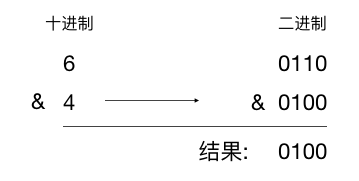& 与：只有当两位都是 1 时结果才是 1，否则为 0 。

``````    0110
&   0100
-----------
0100``````

| 或：两位中只要有 1 位为 1 结果就是 1，两位都为 0 则结果为 0。

``````    0110
&   0110
-----------
0110``````

^ 异或：两个位相同则为 0，不同则为 1

``````    0110
^   0100
-----------
0010``````

~ 取反：0 则变为 1，1 则变为 0

``````~   0110
-----------
1001``````

<< 左移：向左进行移位操作，高位丢弃，低位补 0

``````    int a = 8;
a << 3;

>> 右移：向右进行移位操作，对无符号数，高位补 0，对于有符号数，高位补符号位

``````unsigned     int a = 8;
a >> 3;

int a = -8;
a >> 3;

### 位运算使用技巧简介

1、 判断整型的奇偶性

``````    if((x & 1) == 0) {
// 偶数
}     else {
// 奇数
}``````

2、 判断第 n 位是否设置为 1

``````    if (x & (1<<n)) {
// 第 n 位设置为 1
}
else {
// 第 n 位设置为 1
}``````

3、 将第 n 位设置为 1

``y = x | (1<<n)``

4、 将第 n 位设置为 0

``y = x & ~(1<<n)``

5. 将第 n 位的值取反

``y = x ^ (1<<n)``

``````    01110101
^   00100000
--------
01010101``````

6、 将最右边的 1 设为 0

``y = x & (x-1)``

``````count = 0
while(x) {
x = x & (x - 1);
count++;
}``````

### 巧用位运算解算法题

1、 高频面试题：老鼠试毒

1、 把这 8 个瓶子从 0 到 7 进行编号，用二进制表示如下

``````000
001
010
011
100
101
110
111``````

2、 将 0 到 7 编号中第一位为 1 的所有瓶子（即 1，3，5，7）的水混在一起给老鼠 1 吃，第二位值为 1 的所有瓶子（即2，3，6，7）的水混在一起给老鼠 2 吃， 第三位值为 1 的所有瓶子（4，5，6，7）的水混在一起给老鼠 3 吃，现在假设老鼠 1，3 死了，那么有毒的瓶子编号中第 1，3 位肯定为 1，老鼠 2 没死，则有毒的瓶子编号中第  2 位肯定为 0，得到值 101 ，对应的编号是 5， 也就是第五瓶的水有毒。

2、 leetcode 232

``````00001        2^0 = 1
00010        2^1 = 2
00100        2^2 = 4
01000        2^3 = 8
10000        2^3 = 16``````

``````    if(x&(x-1)) { //使用与运算判断一个数是否是2的幂次方
printf("%d不是2的幂次方！\n", num);
}     else {
printf("%d是2的%d次方！\n", num, log2(num));
}``````

3、 leetcode 232

Python 代码

``````vector<int> countBits(int num) {
vector<int> bits(num+1, 0);
for (int i = 1; i<= num; i++) {
bits[i] += bits[i & (i-1)] + 1;
}
}``````

Java 代码

``````    public    static    int[] countBits(    int num) {
int[] bits =     new    int[num+1];
Arrays.fill(bits, 0);

for (    int i = 1; i <= num; i++) {
bits[i] = bits[i & (i-1)] + 1;
}
return bits;
}``````

``bits[i] += bits[i & (i-1)] + 1;``

4、 利用位运算来解八皇后问题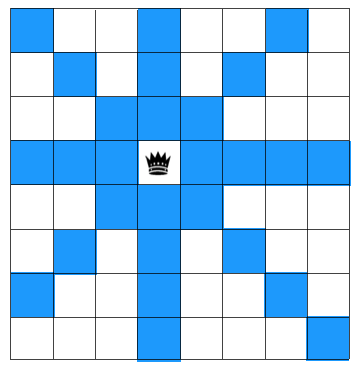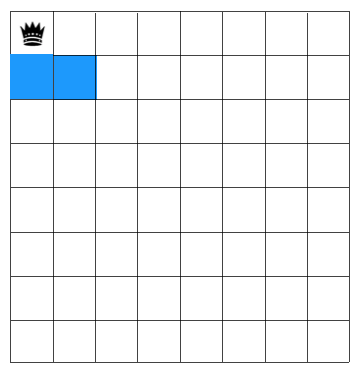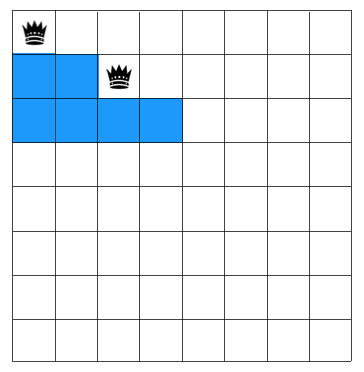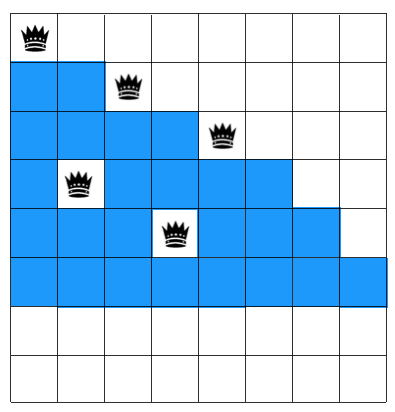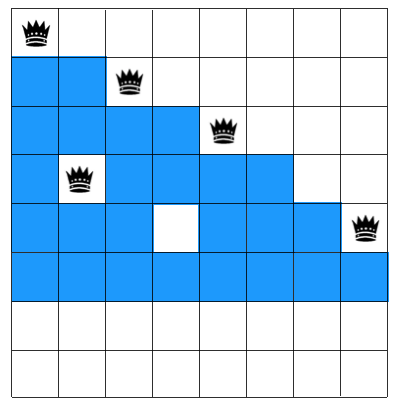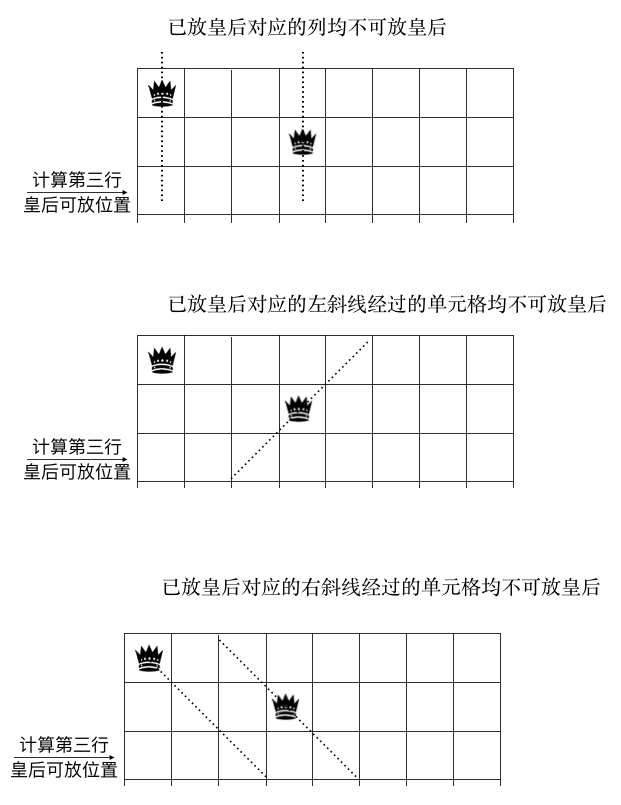``````column = 10010000 (上图中的第一个图，第 1，4 列放了皇后，所以 1，4 位置为 1，其他位置为 0）
pie = 00100000 (上图中的第二个图，左斜线经过第三行的第三个方格，所以第三位为 1）
na = 00101000 (上图中的第三个图，右斜线经过第三行的第三, 五个方格，所以第三,五位为 1）``````

``````    10010000
|   00100000
|   00101000
-----------------------
10111000``````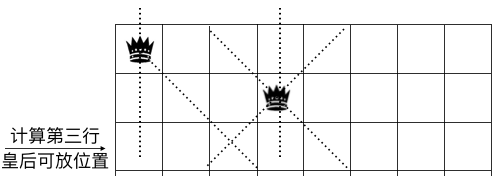``~(column | pie | na) & ((1 << 8)-1)``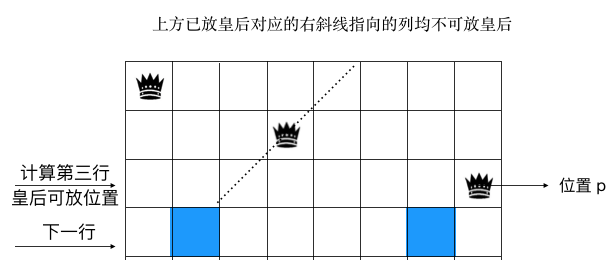``````    void queenSettle(row, colomn,pie,na){
N = 8; // 8皇后
if (row >= N) {
// 遍历到最后一行说明已经找到符合的条件了
count++;    return
}

// 取出当前行可放置皇后的格子
bits = (~(colomn|pie|na)) & ((1 << N)-1)

while(bits > 0) {
// 每次从当前行可用的格子中取出最右边位为 1 的格子放置皇后
p = bits & -bits

// 紧接着在下一行继续放皇后
queenSettle(row+1, colomn | p, (pie|p) << 1, (na | p) >> 1)

// 当前行最右边格子已经选完了，将其置成 0，代表这个格子已遍历过
bits = bits & (bits-1)
}
}``````

### 总结帖子
视频
声望
粉丝
相关问题
社区精华内容